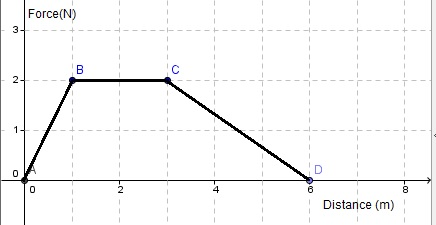# Free SAT II Physics Practice Questions with Solutions Energy, Work and Power

 Sat Physics subject questions on energy, work and power, with detailed solutions, similar to the questions in the SAT test are presented. Answers at the bottom of the page and also detailed solutions and explanations How much work is done in pulling a box, on the ground, for 20 meters with a force of 20 Newtons making an angle of 10� with the ground? A) 400 J B) 4000 J C) 400 tan(10�) J D) 400 cos(10�) J E) 400 sin(10�) J A ball is thrown vertically upward with a velocity of 10 m/s. Calculate the maximum height reached by the ball before it returns to the ground. (g = 10 m/s2) A) 10 m B) 5 m C) 100 m D) 1 m E) 50 m When a car's speed changes from 30 m/s to 15 m/s, its kinetic energy A) is multiplied by 2 B) is divided by 2 C) is divided by 4 D) is multiplied by 4 E) does not change Calculate the work done in pulling a box of 50 Kg up a 20� frictionless incline plane of length 15 m at a constant speed. A) 7500 sin (20�) J B) 7500 J C) 7500 tan (20�) J D) 750 J E) 7500 cos (20�) JFig2. - Force Versus Distance Graph. Approximate the total work done by the force shown in the graph "force versus distance" on the right? A) 12 J B) 6 J C) 2 J D) 4 J E) 8 J A ball is thrown upward at time t1 from the top of a building. At time t2 it reaches a maximum height and heads downward. At time t3 it is at height equal to the height of the building. At time t4, it is at a height equal to half the height of the building. It hits the ground at time t5. At what time is the potential energy of the ball maximum and it kinetic energy equal to zero? A) t1 B) t2 C) t3 D) t4 E) t5 What is the power needed to lift a box of 200 Kg to a height of 20 meters, at a constant speed, in 20 seconds? A) 20,000 w B) 200 w C) 2000 w D) 200,000 w E) 2 w A ball is thrown upward at time t1 from the top of a building. At time t2 it reaches a maximum height and heads downward. At time t3 it is at height equal to the height of the building. At time t4, it is at a height equal to half the height of the building. It hits the ground at time t5. In which interval of time is the potential energy of the ball increasing while its kinetic energy is decreasing? A) t1 , t2 B) t2 , t3 C) t3 , t4 D) t4 , t5 E) t3 , t5 The mass m and velocity v of each of 5 moving objects are given below. Which one has the highest kinetic energy? A) m = 10 Kg , v = 100 m/s B) m = 100 Kg , v = 10 m/s C) m = 1,000 Kg , v = 1 m/s D) m = 10,000 Kg , v = 0.1 m/s E) m = 100,000 Kg , v = 0.01 m/s A forklift lifted a box of mass M at a constant speed to a height of 10 meters using a power of 1000 Watts in 20 seconds. Find mass M. A) 1 Kg B) 2000 Kg C) 20 Kg D) 200 Kg E) 100 Kg Answers to the Above questions D B C A E B C A A D